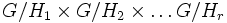# Multicoset for a tuple of subgroups

Let$G$ be a group and$H_1,H_2,\ldots,H_r$ be an$r$-tuple of subgroups of$G$. Consider the natural action of$G$ on the coset space$G/H_1 \times G/H_2 \times \ldots G/H_r$. The orbits under this action are termed the multicosets for the tuple of subgroups.
Multicoset generalizes the notion of left coset and the more general notion of double coset, to a tuple of$r$ subgroups for$r > 2$.